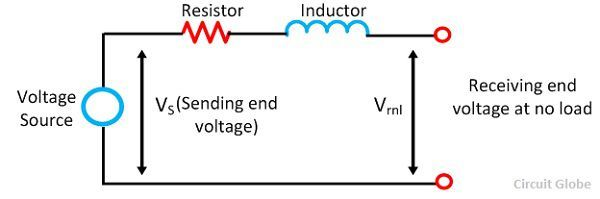# Line Regulation

Definition: The voltage or line regulation is defined as the variation in the voltage at the receiving end of the line when the full load at a given power factor is removed and the voltage at the sending end being kept constant.  In other words, it is defined as the percentage variation in voltage at the load end of the line in going from no load to full load. It is expressed as the fraction of a percentage of the receiving end voltage.The line regulation is given by the equation shown below.where |Vrnl | = magnitude of receiving end voltage at no load.
|Vrfl| = magnitude of receiving end voltage at no load.

The regulation of the line depends on the power factor of the load. For lagging power factor the voltage at the sending end is more than the receiving end of the line. For leading power factor the voltage at the receiving end of the line becomes more than that of the sending end. Because of the leading power factor, the line regulation becomes negative.

The voltage VS at the sending end is kept constant. It is given byWhere Vr0 = 0 is the receiving end voltage at no load.### Line Regulation for Short Line

In the case of the short transmission line, when the load is removed the load at the receiving end is equal to the voltage at the sending end. At full load,

| Vrfl |  = | V|

At no load, |Vrnl | = |Vs|

Therefore, for a short lineThe simplest way of measuring the line regulation is to connect all the three parallel resistors to the supply. The two resistors are attached to the switch while the remaining directly linked to the supply. The value of the resistor chosen in such a way that the resistor which directly connects to the supply has high resistance and the remaining two which are connected in parallel has the nominal value. The voltmeter is placed in parallel with the resistor which measures the voltage at each line which is used for the calculation of line voltage regulation.